• 这里写自定义目录标题欢迎使用Markdown编辑器生成数据作动态图合理的创建标题，有助于目录的生成如何改变文本的样式插入链接与图片 欢迎使用Markdown编辑器 你好！ 这是你第一次使用 Markdown编辑器 所展示的欢迎页...
MATLAB 作动态图MATLAB 作动态图动态线图生成数据作动态图方法一方法二动态点图确定参数生成数据并创建函数句柄迭代以形成动态效果实际应用输出效果
MATLAB 作动态图
本文谨记录笔者探索MATLAB作动态图后的心得，希望能帮到大家
动态线图
生成数据
首先打开MATLAB，生成一组数据。
以下是笔者在练习数学建模中的导弹追踪模型所写的一段代码
由于这段代码涉及的其他知识点较多，也不属于这篇文章的重点，有兴趣的读者可以看看，没有兴趣的可以用后文的替代代码
[x,y]=ode45(@df1,[0:1e-3:0.1],[20 0 0 0],[],200,45);

distance=sqrt((y(:,1)-y(:,3)).^2+(y(:,2)-y(:,4)).^2);
length1=sqrt((y(2:end,3)-y(1:end-1,3)).^2+(y(2:end,4)-y(1:end-1,4)).^2);
length1=cumsum(length1);
choose=min(find(distance<=1e-1))-1;
length1(choose)

plot(y(:,1),y(:,2),y(:,3),y(:,4),y(choose,1),y(choose,2),'bo',y(choose,3),y(choose,4),'ro')
% axis([0,max(y(:,1)),0,max(y(:,1))]

function dy=df1(t,y,v,si)
%     v=200;
dy=zeros(4,1);
dy(1)=cos(si/180*pi)*v;
dy(2)=sin(si/180*pi)*v;
dy(3)=3*v*(y(1)-y(3))/sqrt((y(1)-y(3))^2+(y(2)-y(4))^2);
dy(4)=3*v*(y(2)-y(4))/sqrt((y(1)-y(3))^2+(y(2)-y(4))^2);
end

由于笔者使用的是实时脚本（听说是MATLAB 2016a之后才有），其可以使用局部函数，只需写在实时脚本最后即可。
而若使用普通脚本，则需在当前工作路径下新建函数文件，将其放入其中即可。
方便起见，使用普通脚本也可用下列代码代替
clear;clc
x=1:200;
y1=0.01*x;
y2=x-100;
y=[x;y1;x;y2]';
choose=100;

作动态图
方法一
有了数据之后便可以开始作图了，以下便是作图代码
for i = 1 : choose
plot(y(i:i+1,1),y(i:i+1,2),'b','MarkerSize',1)
plot(y(i:i+1,3),y(i:i+1,4),'r','MarkerSize',1)
hold on
axis([min(y(:,3)),max(y(:,3)),min(y(:,4)),max(y(:,4))]);
pause(0.1)
end

可以看到，这段代码先是逐段画线，然后用hold on保持图像，用pause控制画线速度，用choose控制画线的终点，这里取的是两条线的交点。Fig 1.这是原代码画出来的线Fig 2.这是替代代码画出来的线
方法二
实际上，在MATLAB 2016 及之后的版本，其新增了一个画动态线图的函数，使用起来比方法一要方便

函数方法
说明
输入参数

animatedline
创建动态线图的函数句柄
(无输入参数)

addpoints(h,x,y)
往动态线图新增点
(动态线图的句柄，点的x坐标，点的y坐标)

getpoints(h)
获取动态线图的所有点
(动态线图的句柄）

clearpoints(h)
清除动态线图的所有点
(动态线图的句柄）

h=animatedline;
axis([0 4*pi -1 1])
x=linspace(0,4*pi,1000);
y=sin(x);
for k = 1:length(x)
addpoints(h,x(k),y(k));
pause(0.01)
end

动态点图
确定参数
先确定几个参数
n = 10; % 粒子数量
K = 10;  % 迭代的次数
vmax = [6 6]; % 粒子的最大速度
x_lb = [-15 -15]; % x的下界
x_ub = [15 15]; % x的上界

生成数据并创建函数句柄
然后笔者给出了一个实例函数，作出二维网格后计算出各点的值然后绘制出网格
其中 view([-39 45]) 是笔者认为可以较好展示此网格的角度
x1=x_lb(1):x_ub(1);
x2=x_lb(2):x_ub(2);
[x1,x2]=meshgrid(x1,x2);
y = x1.^2 + x2.^2 - x1.*x2 - 10*x1 - 4*x2 + 60;
mesh(x1,x2,y)
view([-39 45])
hold on

迭代以形成动态效果
然后迭代K次，给予一个随机数让点动起来，然后用一个for循环将点限制在绘图所在区域
然后利用函数句柄绘制散点图，即可动态展示散点
此处为了方便，用了一个函数（在实时脚本中放在最后即可，无需新建函数文件），也可用上一段代码的y来代替，其输出效果放在了实际应用后面
for d = 1:K
x=x-vmax+2*vmax.*rand(n,narvs);
for i = 1:narvs
x(x(:,i)<x_lb(i),i)=x_lb(i);
x(x(:,i)>x_ub(i),i)=x_ub(i);
end

pause(0.5);
h.XData=x(:,1);
h.YData=x(:,2);
h.ZData=Obj_fun1(x);
end

hold off

function y=Obj_fun1(x)
y=x(:,1).^2+x(:,2).^2-x(:,1).*x(:,2)-10*x(:,1)-4*x(:,2)+60;
end

实际应用
这其实是笔者在写粒子群算法时想到的一种动态展示散点图的方式，以下是全部代码
%% 粒子群算法（最小值）
%% 设置粒子群算法的参数
n = 10; % 粒子数量
narvs = 2; % 变量个数
c1 = 2;  % 每个粒子的个体学习因子，也称为个体加速常数
c2 = 2;  % 每个粒子的社会学习因子，也称为社会加速常数
w = 0.9;  % 惯性权重
K = 10;  % 迭代的次数
vmax = [6 6]; % 粒子的最大速度
x_lb = [-15 -15]; % x的下界
x_ub = [15 15]; % x的上界

%% 初始化粒子的位置和速度
x=x_lb+(x_ub-x_lb).*rand(n,narvs);
v=-vmax+2*vmax.*rand(n,narvs);

%% 计算适应度
fit=Obj_fun1(x);
pbest=x;
ind=find(fit==min(fit));
gbest=x(ind,:);

%% 画图
x1=x_lb(1):x_ub(1);
x2=x_lb(2):x_ub(2);
[x1,x2]=meshgrid(x1,x2);
y = x1.^2 + x2.^2 - x1.*x2 - 10*x1 - 4*x2 + 60;
mesh(x1,x2,y)
view([-39 45])
hold on
h=scatter3(x(:,1),x(:,2),fit,'*r');

%% 更新粒子速度和位置
gbest_val_list=zeros(1,K);
for d = 1:K
v=w*v+c1*rand(n,narvs).*(pbest-x) ...
+c2*rand(n,narvs).*(gbest-x);
for i = 1:narvs
v(v(:,i)<-vmax(i),i)=-vmax(i);
v(v(:,i)>vmax(i),i)=vmax(i);
end
x=x+v;
for i = 1:narvs
x(x(:,i)<x_lb(i),i)=x_lb(i);
x(x(:,i)>x_ub(i),i)=x_ub(i);
end
fit=Obj_fun1(x);
pbest(fit<Obj_fun1(pbest),:)=x(fit<Obj_fun1(pbest),:);
if sum(Obj_fun1(pbest)<Obj_fun1(gbest)) > 0
gbest=pbest(Obj_fun1(pbest) == min(Obj_fun1(pbest)),:);
end
gbest_val_list(d)=Obj_fun1(gbest);

pause(0.5);
h.XData=x(:,1);
h.YData=x(:,2);
h.ZData=fit;
end

%% 输出
hold off
plot(1:K,gbest_val_list)
disp('最优解坐标为:')
disp(gbest)
disp('最优解值为:')
disp(Obj_fun1(gbest))

function y=Obj_fun1(x)
y=x(:,1).^2+x(:,2).^2-x(:,1).*x(:,2)-10*x(:,1)-4*x(:,2)+60;
end

输出效果Fig 3.起始点的分布情况Fig 4.粒子群算法运行完毕时各点的分布情况
可以看到，这样的展示方式可以直观地看出粒子群算法寻找函数最小值的过程
本文到此结束，如有啥问题，可以留言告诉我哦


展开全文• 使用Matlab制作动画-MATLAB_动画图_____作动态图.pdf Matlab制作动画的几种方法。
• ## MATLAB 制作gif动态图

万次阅读 多人点赞 2017-12-20 22:26:25
MATLAB 绘制gif动态图的基本思想就是，将一张张的静态图组合成一张能动的gif图片，并保存到相应的位置。 那么，要想制作一张动态图，首先要有若干个静态图，并且他们的索引值是连续的。下面先说一下matlab关于静态...
MATLAB 制作gif动态图的基本思想就是，将一张张的静态图组合成一张能动的gif图片，并保存到相应的位置。
那么，要想制作一张动态图，首先要有若干个静态图，并且他们的索引值是连续的。下面先说一下matlab关于静态图的绘制及其保存。

1、MATLAB静态图绘制及保存

下面以一个sin函数为例进行说明。实例是这样的：有一个运动轨迹sin(t),t=0:30。现在有一个小球去跟踪这个sin曲线。
（1）首先画出y=sin(t)的图像：
m语言：

t=1:0.1:30;
y=sin(t);
plot(t,y);
grid on


得到如下图像：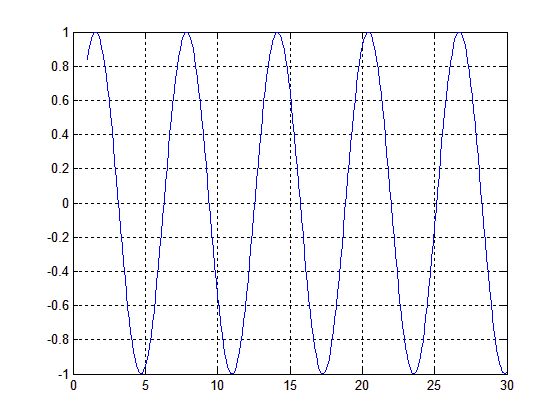接下来要定义一个小球去跟踪上面的曲线，并将跟踪过程中生成的图片保存到当前工作路径下的image文件夹下：

%迭代值
i=0;
%设置小球属性，横坐标数据为'xdata',纵坐标数据为'ydata',红色，大小为30
h = line('xdata',[],'ydata',[],'color','r','marker','.','markersize',30);
for ii=1:length(y)
%跟踪上述曲线
set(h,'xdata',t(ii),'ydata',y(ii));
%建立新图像
figure();
%绘制
drawnow
%可认为是小球一定速度
pause(0.1)
hold on
i=i+1;
%保存图片，位置为:/image，名称为i.bmp
print(1,'-dbmp',sprintf('image/%d',i))
%关闭figure()
close;
end

由此变生成了0.bmp~291.bmp共291张图片，并保存在当前工作路径下的image文件夹下，如下图所示。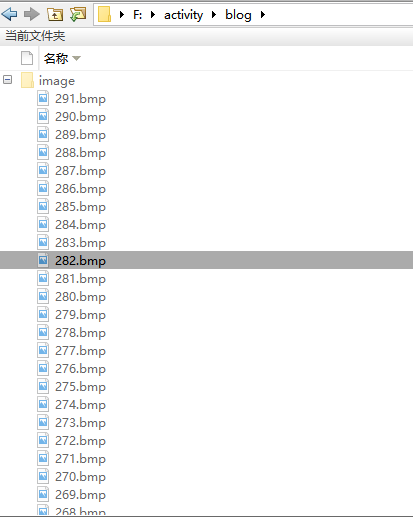2、生成gif图片

根据上述操作，我们得到了名为0-291.bmp的图片。接下来要根据这291张图片制作一张gif图片。

%依次读取生成的所有图片
for j=1:length(y)
%获取当前图片
A=imread(sprintf('image/%d.bmp',j));
[I,map]=rgb2ind(A,256);
%生成gif，并保存
if(j==1)
imwrite(I,map,'movefig.gif','DelayTime',0.1,'LoopCount',Inf)
else
imwrite(I,map,'movefig.gif','WriteMode','append','DelayTime',0.1)
end
end

最后生成的gif图片如下：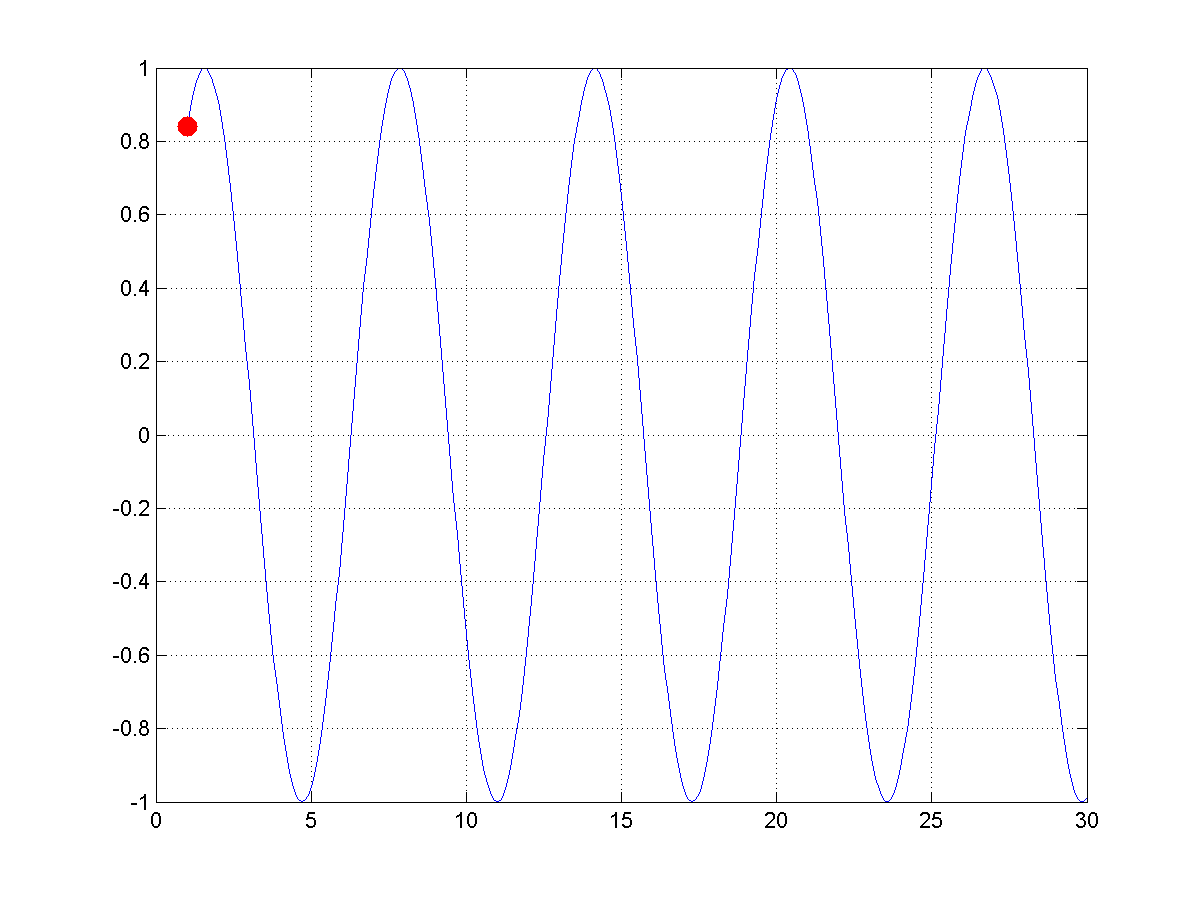展开全文• ## Matlab制作GIF动态图

万次阅读 2018-05-24 14:22:33
GIF动态图制作制作 clc clear close all filename='D:\\matlab_program\\Mycode\\fish1.gif'; % 输出路径+保存的文件名.gif for i=3374:3379 str = sprintf('D:\\matlab_program\\Mycode\\%d.png',i); %图片绝对...
GIF动态图制作制作

/**使用这个程序只需将循环中的i=3374:3379改成自己的图像的编号即可，还有str=sprintf()中的图片的类型也要修改**/
clc
clear
close all
filename='D:\\matlab_program\\Mycode\\fish1.gif';          %输出路径+保存的文件名.gif
for i=3374:3379
str = sprintf('D:\\matlab_program\\Mycode\\%d.png',i); %图片绝对位置和类型，注意这里的图片类型不能是bmp格式
img = imread(str);     %读取图像
figure(i)
imshow(img)
set(gcf,'color','w');  %设置背景为白色
set(gca,'units','pixels','Visible','off');
frame = getframe(gcf);
im = frame2im(frame);     %将影片动画转换为编址图像,因为图像必须是index索引图像
imshow(im);
[I,map] = rgb2ind(im,20); %将真彩色图像转化为索引图像
if i==3374;
imwrite(I,map,filename,'gif','Loopcount',inf,'DelayTime',0.3);     %Loopcount只是在i==1的时候才有用
else
imwrite(I,map,filename,'gif','WriteMode','append','DelayTime',0.3);%DelayTime:帧与帧之间的时间间隔
end
end
close all

＝＝＝＝一条快乐的分割线＝＝＝＝

代码帮助

set(gca,'units','pixels','Visible','off');
/**
Units：有效值为pixels/normalized/inches /centimeters/points/characters
决定各种位置属性的度量单位，相对于窗口左下角为基准。当设置为Pixels时，以像素为单位；
当设置为normalized时，以坐标为单位，屏幕的左下角为[0，0]，右上角为[1.0，1.0]。
Visible:决定坐标轴是否可见
**/

[X,map] = rgb2ind(RGB,n)
/**
使用最小方差量化和抖动将RGB图像转换为索引图像X。map最多包含n个颜色。
**/

MATLAB getframe用法

F = getframe(H,Rect) 

H：图像句柄
Rect：一个四元素向量，Rect=[a b c d]，其中a表示截取区域到图像左端的像素数，b表示截取区域到图像底端的像素数，c表示截取区域水平像素数，d表示截取区域垂直向像素数。
F：是一个结构体,包含两个元素：cdata和colormap
cdata：Rect中参数选择的区域的数据，一个三维数组，其中第三维的长度是3，即截取到的是区域中RGB的值。
colormap：调色板数据

效果图对比

当[X,map] = rgb2ind(RGB,n) %n=256时 由于图像是以8位存储的，n最大等于256。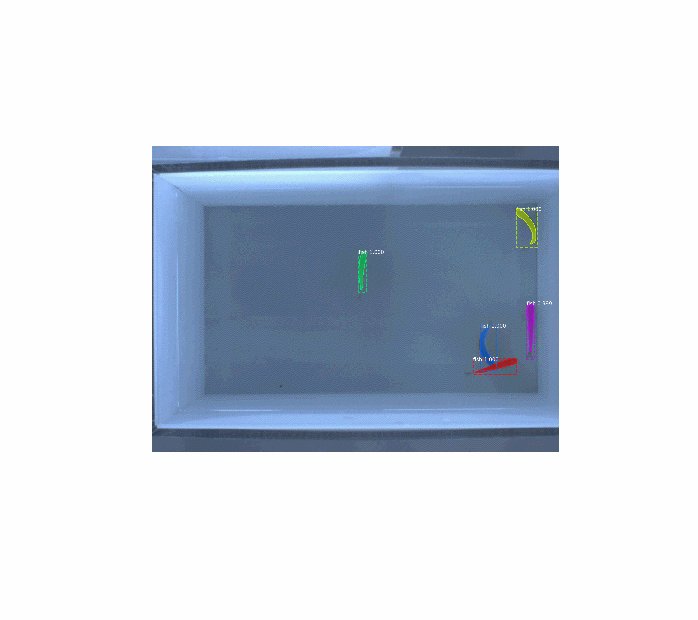当[X,map] = rgb2ind(RGB,n) %n=20时，可以看到n=20时，图片明显的模糊了很多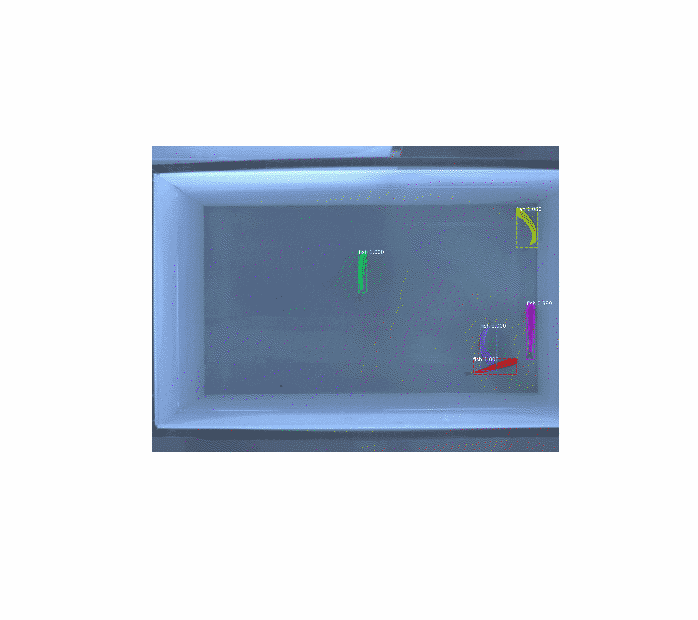当[X,map] = rgb2ind(RGB,n) %n=0时，可以看到n=0时，图片丢失了更多的信息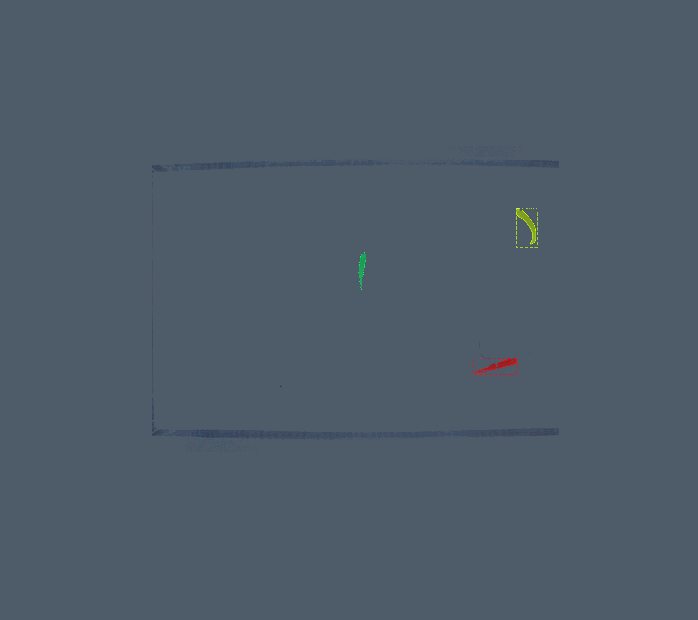展开全文图片合成
• 主要介绍了Matlab制作视频并转换成gif动态图的两种方法,第一种方法使用movie（f）直接取生成AVI视频文件，相对来说比较简单，需要的朋友可以参考下
• 动图一般有两种格式.gif以及.avi，都能拥有更好的展示效果 %% avi close all; clear all; aviobj = VideoWriter('test.avi','Uncompressed AVI'); open(aviobj) t = linspace(0,2.5*pi,40);...[x,y,z] = pea...
动图一般有两种格式.gif以及.avi，都能拥有更好的展示效果
%% avi
close all; clear all;

aviobj = VideoWriter('test.avi','Uncompressed AVI');
open(aviobj)

t = linspace(0,2.5*pi,40);
fact = 10*sin(t);
fig=figure;
[x,y,z] = peaks;
for k=1:length(fact)
h = surf(x,y,fact(k)*z);
axis([-3 3 -3 3 -80 80])
axis off
caxis([-90 90])
F = getframe(fig);
writeVideo(aviobj,F);
im = frame2im(F);
[I,map] = rgb2ind(im,256);
if k == 1
imwrite(I,map,'test1.gif','GIF', 'Loopcount',inf,'DelayTime',0.1);
else
imwrite(I,map,'test1.gif','GIF','WriteMode','append','DelayTime',0.1);
end
end
close(fig);
close(aviobj);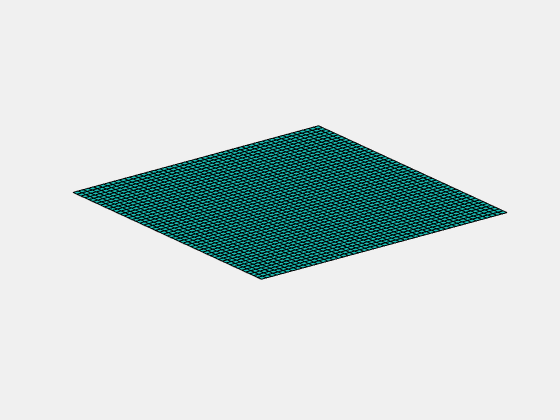%% gif
clear all
h = animatedline;%动画线
axis([0 4*pi -1 1])
box on
x = linspace(0,4*pi,200);

for k = 1:length(x)
y = sin(x(k));
addpoints(h,x(k),y);%将数据添加到动画线中
drawnow%画出动画线
f=getframe(gcf);
imind=frame2im(f);
[imind,cm] = rgb2ind(imind,256);
if k == 1
imwrite(imind,cm,'test.gif','GIF', 'Loopcount',inf,'DelayTime',1);
else
imwrite(imind,cm,'test.gif','GIF','WriteMode','append','DelayTime',1);
end
end
甚至也可以将.tif文件用这种方法做成动图展开全文动图
• 第一种方法用movie(f)直接生成 %% f(t)-->f(4*t+12) 并且验证%% function Signal_change() tic%记录程序运行时间 figure n = 0; t = -2*pi:0.01:2*pi; y = sin(t);%周期为2*pi y_result = sin(4*t);...ylabel('...
• 动态窗的口法（Dynamic Window Approach，DWA）与Matlab中gif动态图的制作 动态窗口方法是由Dieter Fox，Wolfram Burgard和Sebastian Thrun在1997年开发的用于移动机器人在线避障的一种策略。动态窗口法根据机器人...
• MATLAB如何制作动画（动态图形演示movie） 听语音 | 浏览：7100 | 更新：2017-12-10 10:57 | 标签：MATLAB动画 1 2 3 4 5 6 7 分步...制作动画
• 前面用matlab制作了gif的动态心形曲线：matlab制作动态心形曲线（保存为gif版本） % matlab动态心形曲线（AVI视频版本） clc;clear all; a=0; x=-2:0.01:2; writerObj = VideoWriter('心形.avi'); writerObj.Frame...
• MATLAB movie 函数动态绘图 电影动画的好处就是，运行一次可以...step1：调用moviein函数对内存进行初始化(该步骤在Matlab5.3以上均可省略)，创建一个足够大的矩阵，使之能够容纳基于当前坐标轴大小的一系列指定的
• 脚本之家你与百万开发者在一起作者：theOwlAndPussyCat/焦旭光引言电脑里存了很多有意思的Gif动态图片，闲暇想把这些动图全导入微信表情，可是这些动图很多大小超过了微信表情大小1MB的限制，要制作成表情只能压缩...# matlab作动态图matlab 订阅